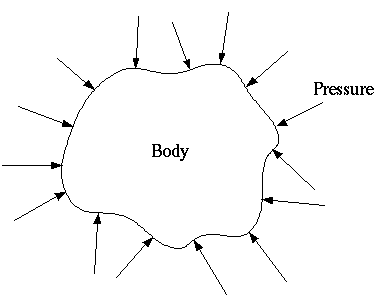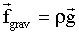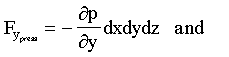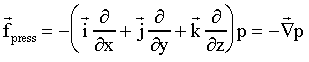### Introduction to Pressure in Fluid Mechanics

• Pressure always acts inward normal to any surface (even imaginary surfaces as in a control volume).• Pressure is a normal stress, and hence has dimensions of force per unit area, or {ML-1T-2}. In the English system of units, pressure is expressed as "psi" or lbf/in2. In the Metric system of units, pressure is expressed as "pascals" or N/m2.
• Standard atmospheric pressure is 101.3 kPa (0.1013 MPa) or 14.69 psi.
Pressure Terminology
There are three different kinds of pressure reported in the literature, and it is important to know the terminology:
• Absolute pressure is measured relative to absolute zero on the pressure scale, which is a perfect vacuum. (Absolute pressure can never be negative.) Absolute pressure is indicated by p, and is identical to the familiar thermodynamic pressure.
• Gage pressure (sometimes written as "gauge pressure") is measured relative to the local atmospheric pressure. Gage pressure is thus zero when the pressure is the same as atmospheric pressure. (It is possible to have negative gage pressure.) Gage pressure is indicated by pg, and is related to absolute pressure as follows: pg = p - pa, where pa is the local atmospheric pressure.
• Example: A car tire gauge measures a tire pressure of 32.0 psi. The local atmospheric pressure is 14.2 psi. What is the absolute pressure of the air in the tire?
Solution: The tire gauge measures gage pressure, relative to the local value of atmospheric pressure. Thus, p = pg + pa = 32.0 + 14.2 psi = 46.2 psia. Note that in the English system, absolute pressure in psi is often denoted "psia", while gage pressure in psi is often denoted "psig". There is no such distinction in the metric system.
• Example: Does gage pressure change with elevation?
Solution: No. Since gage pressure is always relative to the local value of atmospheric pressure, the gage pressure of the atmosphere is always zero, regardless of elevation. Note that absolute pressure decreases with elevation, just as water pressure increases with depth.
• Vacuum pressure is also measured relative to the local atmospheric pressure, but is used when the gage pressure is negative, i.e. when the absolute pressure falls below the local atmospheric pressure. (Positive vacuum pressure means that the gage pressure is negative.) Vacuum pressure is indicated by pvacuum, and is related to absolute pressure as follows: pvacuum = pa - p, where pa is the local atmospheric pressure.

Equations for pressure distribution in a fluid

 Consider a small fluid element of dimensions dx, dy, and dz as a free body diagram, on which all forces acting on the element will be discussed. There are three kinds of forces important to fluid mechanics: gravity (body force), pressure forces, and viscous forces (due to friction). Gravity force,is shown in the sketch. Note the convention that gravity acts in the minus z direction.• Imagine the fluid element shrinking to a point (dx, dy, and dz all go to zero). The net force on the element would then go to zero. Instead of force, then, it is more relevant to consider force per unit volume, which will be denoted by a lower case f. For gravity, then,is the gravity force per unit volume acting on the element.
• Next, consider the net pressure force. At each of the six faces of the fluid element, an average pressure will act normal and inward on the face. Since p is a field variable, i.e. p = p(x,y,z,t) in general, the average pressure on one side of the face will not necessarily be identical to that on the opposite face. For example, consider the pressures acting on the two faces which lie perpendicular to the x-axis, i.e. the pressure forces in the x direction, as shown in the sketchA Taylor series (truncated to first order) is used to account for the change in pressure in the x direction over the short distance dx. The net component of pressure force on the element in the x direction iswhere since pressure is a stress (force per unit area), pressure must be multiplied by the area of the face (dydz) to get dimensions of force. Two of the terms in the above expression cancel, and• Similarly, the net pressure forces in the y and z directions areOf interest here is the net vector pressure force per unit volume. Dividing by the volume of the element (dxdydz) and writing these three components as a single vector leads towhere the right hand side is the negative of the gradient of pressure. Thus it can be noted that it is not pressure itself which causes a net pressure force, but rather pressure gradients. In other words, there is no net pressure force on a fluid element unless pressure changes from one face of the element to another.
• In cases where there is no fluid motion, the only forces acting on a fluid element are the body force (gravity) and the forces due to pressure. Since there is no acceleration, summation of all forces on the element must equal zero; pressure forces are exactly balanced by gravity forces. This situation is known as fluid statics or hydrostatics.